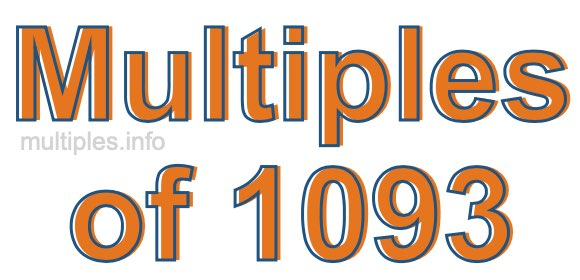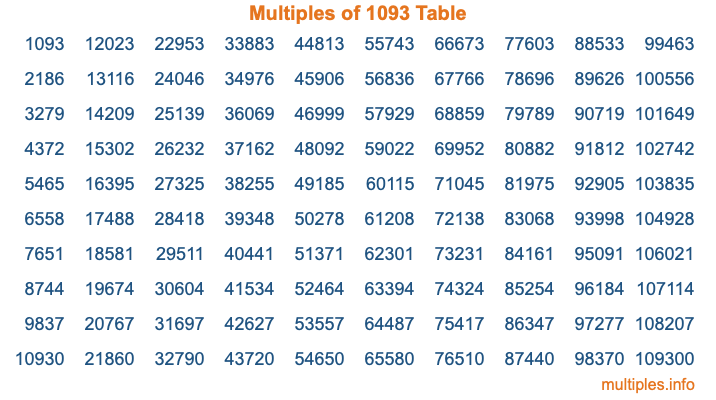Multiples of 1093Welcome to the Multiples of 1093 page. Here we will first teach you everything you will ever need to know about the multiples of 1093, and then give you a study guide summary of everything we taught you to make sure you remember it all. Use this page to look up facts and learn information about the multiples of 1093. This page will make you a multiples of one thousand ninety-three expert!

Definition of Multiples of 1093
Multiples of 1093 are all the numbers that when divided by 1093 equal an integer. Each of the multiples of 1093 are called a multiple. A multiple of 1093 is created by multiplying 1093 by an integer.

Therefore, to create a list of multiples of 1093, you start with 1 multiplied by 1093, then 2 multiplied by 1093, then 3 multiplied by 1093, and so on for as long as you want. Thus, the list of the first five multiples of 1093 is 1093, 2186, 3279, 4372, and 5465. To see a larger list of multiples of 1093, see the printable image of Multiples of 1093 further down on this page. We also have a category where you can choose any nth multiple of 1093.

Multiples of 1093 Checker
The Multiples of 1093 Checker below checks to see if any number of your choice is a multiple of 1093. In other words, it checks to see if there is any number (integer) that when multiplied by 1093 will equal your number. To do that, we divide your number by 1093. If the the quotient is an integer, then your number is a multiple of 1093.

Is  a multiple of 1093?

Least Common Multiple of 1093 and ...
A Least Common Multiple (LCM) is the lowest multiple that two or more numbers have in common. This is also called the smallest common multiple or lowest common multiple and is useful to know when you are adding our subtracting fractions. Enter one or more numbers below (1093 is already entered) to find the LCM.

Check out our LCM Calculator if you need more details about the Least Common Multiple or if you need the LCM for different numbers for adding and subtraction fractions.

nth Multiple of 1093
As we stated above, 1093 is the first multiple of 1093, 2186 is the second multiple of 1093, 3279 is the third multiple of 1093, and so on. Enter a number below to find the nth multiple of 1093.

th multiple of 1093

Multiples of 1093 vs Factors of 1093
1093 is a multiple of 1093 and a factor of 1093, but that is where the similarities end. All postive multiples of 1093 are 1093 or greater than 1093. All positive factors of 1093 are 1093 or less than 1093.

Below is the beginning list of multiples of 1093 and the factors of 1093 so you can compare:

Multiples of 1093: 1093, 2186, 3279, 4372, 5465, etc.

Factors of 1093: 1, 1093

As you can see, the multiples of 1093 are all the numbers that you can divide by 1093 to get a whole number. The factors of 1093, on the other hand, are all the whole numbers that you can multiply by another whole number to get 1093.

It's also interesting to note that if a number (x) is a factor of 1093, then 1093 will also be a multiple of that number (x).

Multiples of 1093 vs Divisors of 1093
The divisors of 1093 are all the integers that 1093 can be divided by evenly. Below is a list of the divisors of 1093.

Divisors of 1093: 1, 1093

The interesting thing to note here is that if you take any multiple of 1093 and divide it by a divisor of 1093, you will see that the quotient is an integer.

Multiples of 1093 Table
Below is an image of the first 100 multiples of 1093 in a table. The table is in chronological order, column by column. The first column has the first ten multiples of 1093, the second column has the next ten multiples of 1093, and so on.The Multiples of 1093 Table is also referred to as the 1093 Times Table or Times Table of 1093. You are welcome to print out our table for your studies.

Negative Multiples of 1093
Although not often discussed or needed in math, it is worth mentioning that you can make a list of negative multiples of 1093 by multiplying 1093 by -1, then by -2, then by -3, and so on, to get the following list of negative multiples of 1093:

-1093, -2186, -3279, -4372, -5465, etc.

Multiples of 1093 Summary
Below is a summary of important Multiples of 1093 facts that we have discussed on this page. To retain the knowledge on this page, we recommend that you read through the summary and explain to yourself or a study partner why they hold true.

There are an infinite number of multiples of 1093.

A multiple of 1093 divided by 1093 will equal a whole number.

1093 divided by a factor of 1093 equals a divisor of 1093.

The nth multiple of 1093 is n times 1093.

The largest factor of 1093 is equal to the first positive multiple of 1093.

1093 is a multiple of every factor of 1093.

1093 is a multiple of 1093.

A multiple of 1093 divided by a divisor of 1093 equals an integer.

1093 divided by a divisor of 1093 equals a factor of 1093.

Any integer times 1093 will equal a multiple of 1093.

Multiples of a Number
Here you can get the multiples of another number, all with the same attention to detail as we did for multiples of 1093 on this page.

Multiples of
Multiples of 1094
Did you find our page about multiples of one thousand ninety-three educational? Do you want more knowledge? Check out the multiples of the next number on our list!